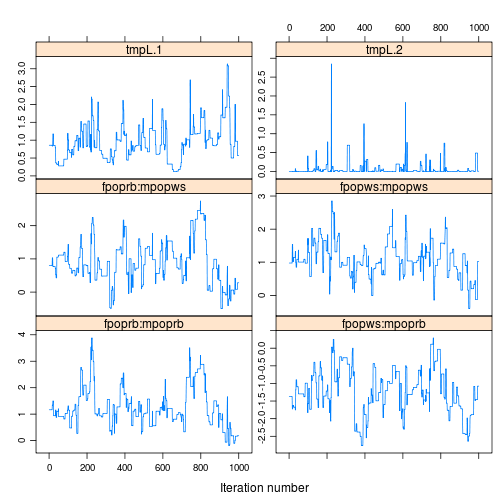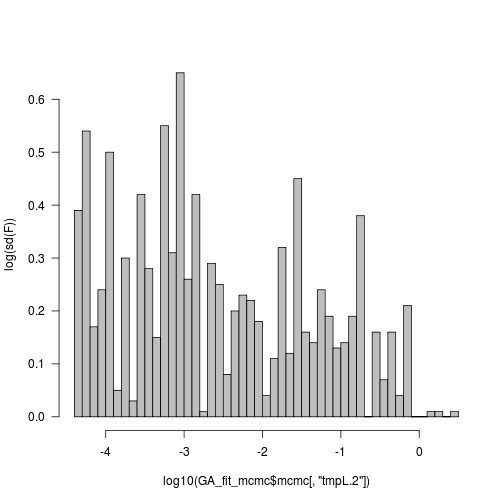# Salamander GLMM

The “salamander” example is a classic example in generalized linear mixed models (e.g. see this example from Charlie Geyer, or this one from the SAS PROC GLIMMIX documentation). Geyer points out that this example has been discussed by:

McCullagh and Nelder (1989), Schall (1991), Karim and Zeger (1992), Breslow and Clayton (1993), Wolfinger and O’Connell (1993), and Shun (1997).

(More specifically, it has been covered by @booth_maximizing_1999 and by @sung_monte_2007.)

Estimates of female and male variance parameters from SAS PROC GLIMMIX:

``````##      var_est var_se
## fnum 1.40990 0.8871
## mnum 0.08963 0.4102
``````

Estimates of all parameters (from @booth_maximizing_1999 by way of Geyer's example).

``````##     R/R     R/W     W/R     W/W sigma_f sigma_m
##   1.030   0.320  -1.950   0.990   1.183   1.118
``````

Geyer considers these results to be the gold standard (he gets close to them with a sufficiently thorough Monte Carlo maximum likelihood fit, and says he has independently confirmed with an MCMC fit). As shown below, it seems as though most available algorithms estimate the among-female variance by quite a lot (orders of magnitude, although surprisingly (to me) SAS PROC GLIMMIX is one of the closest …

``````sdat <- read.table("salamander.txt", header = TRUE, colClasses = c(rep("factor",
5), "numeric"))
``````
``````with(sdat, table(fpop, mpop))
``````
``````##     mpop
## fpop rb ws
##   rb 30 30
##   ws 30 30
``````
``````with(sdat, table(fnum, mnum))
``````
``````##     mnum
## fnum 1 10 2 3 4 5 6 7 8 9
##   1  2  2 0 0 2 2 0 1 1 2
##   10 0  1 2 2 1 1 1 2 2 0
##   2  1  1 1 2 0 2 2 2 0 1
##   3  2  0 1 2 1 0 2 2 1 1
##   4  0  2 2 0 2 2 0 0 2 2
##   5  1  1 2 2 1 0 2 1 2 0
##   6  1  1 2 1 1 1 1 1 1 2
##   7  2  0 1 0 1 2 1 1 2 2
##   8  1  2 1 1 1 2 2 1 0 1
##   9  2  2 0 2 2 0 1 1 1 1
``````
``````library(lme4)
glmer(mating ~ 0 + fpop:mpop + (1 | mnum) + (1 | fnum), sdat, family = binomial)
``````
``````## Generalized linear mixed model fit by maximum likelihood ['glmerMod']
##  Family: binomial ( logit )
## Formula: mating ~ 0 + fpop:mpop + (1 | mnum) + (1 | fnum)
##    Data: sdat
##
##      AIC      BIC   logLik deviance
##    148.8    165.5    -68.4    136.8
##
## Random effects:
##  Groups Name        Variance Std.Dev.
##  mnum   (Intercept) 7.35e-01 8.57e-01
##  fnum   (Intercept) 1.88e-10 1.37e-05
## Number of obs: 120, groups: mnum, 10; fnum, 10
##
## Fixed effects:
##               Estimate Std. Error z value Pr(>|z|)
## fpoprb:mpoprb    1.165      0.515    2.26    0.024 *
## fpopws:mpoprb   -1.371      0.533   -2.57    0.010 *
## fpoprb:mpopws    0.800      0.490    1.63    0.103
## fpopws:mpopws    0.978      0.501    1.95    0.051 .
## ---
## Signif. codes:  0 '***' 0.001 '**' 0.01 '*' 0.05 '.' 0.1 ' ' 1
##
## Correlation of Fixed Effects:
##             fpprb:mppr fppws:mppr fpprb:mppw
## fppws:mpprb 0.258
## fpprb:mppws 0.298      0.274
## fppws:mppws 0.293      0.267      0.305
``````
``````library(glmmADMB)
GA_fit0 <- glmmadmb(mating ~ 0 + fpop:mpop + (1 | mnum) + (1 | fnum), sdat,
family = "binomial")
``````

Using importance sampling to try to do better (see D. Fournier's post):

``````## 25 points, 50 samples, random number seed=121:
GA_fit_is1 <- glmmadmb(mating ~ 0 + fpop:mpop + (1 | mnum) + (1 | fnum), sdat,
family = "binomial", extra.args = "-is 25  121  50")
GA_fit_is2 <- glmmadmb(mating ~ 0 + fpop:mpop + (1 | mnum) + (1 | fnum), sdat,
family = "binomial", extra.args = "-is 50  121  100")
``````

Conclusion: Results are similar from both approaches; Laplace approximation doesn't work that well.

Try MCMC?

``````GA_t_mcmc <- system.time(GA_fit_mcmc <- glmmadmb(mating ~ 0 + fpop:mpop + (1 |
mnum) + (1 | fnum), sdat, family = "binomial", mcmc = TRUE))
``````
``````## Loading required package: coda
``````

Diagnose (the `tmpL` variables represent the variance parameters, on a standard deviation scale).

``````library(coda)
xyplot(GA_fit_mcmc\$mcmc[, 1:6], layout = c(2, 3), aspect = "fill")
``````A close-up of the female-sd parameter:Bottom line, we would have to do significantly more fussing to get a good answer this way.

glmmPQL should in principle work, but http://tolstoy.newcastle.edu.au/R/help/05/01/10005.html

``````library(MASS)
library(nlme)
## http://tolstoy.newcastle.edu.au/R/help/05/01/10005.html
sdat\$dummy <- factor(rep(1, nrow(sdat)))
sdatG <- groupedData(mating ~ 1 | dummy, sdat)
glmmPQL(mating ~ 0 + fpop:mpop, data = sdatG, random = pdBlocked(list(pdIdent(~mnum -
1), pdIdent(~fnum - 1))), family = binomial)
``````
``````## Error: invalid formula for groups
``````
``````## this one works, but only with groupedData
lme(mating ~ 0 + fpop:mpop, data = sdatG, random = pdBlocked(list(pdIdent(~mnum -
1), pdIdent(~fnum - 1))))
``````
``````## Linear mixed-effects model fit by REML
##   Data: sdatG
##   Log-restricted-likelihood: -77.46
##   Fixed: mating ~ 0 + fpop:mpop
## fpoprb:mpoprb fpopws:mpoprb fpoprb:mpopws fpopws:mpopws
##        0.7333        0.2333        0.6667        0.7000
##
## Random effects:
##  Composite Structure: Blocked
##
##  Block 1: mnum1, mnum10, mnum2, mnum3, mnum4, mnum5, mnum6, mnum7, mnum8, mnum9
##  Formula: ~mnum - 1 | dummy
##  Structure: Multiple of an Identity
##          mnum1 mnum10  mnum2  mnum3  mnum4  mnum5  mnum6  mnum7  mnum8
## StdDev: 0.1673 0.1673 0.1673 0.1673 0.1673 0.1673 0.1673 0.1673 0.1673
##          mnum9
## StdDev: 0.1673
##
##  Block 2: fnum1, fnum10, fnum2, fnum3, fnum4, fnum5, fnum6, fnum7, fnum8, fnum9
##  Formula: ~fnum - 1 | dummy
##  Structure: Multiple of an Identity
##             fnum1    fnum10     fnum2     fnum3     fnum4     fnum5
## StdDev: 4.901e-05 4.901e-05 4.901e-05 4.901e-05 4.901e-05 4.901e-05
##             fnum6     fnum7     fnum8     fnum9 Residual
## StdDev: 4.901e-05 4.901e-05 4.901e-05 4.901e-05   0.4273
##
## Number of Observations: 120
## Number of Groups: 1
``````
``````data(Assay)  ## from PB p 165
Assay <- as.data.frame(Assay)
fm1Assay <- lme(logDens ~ sample * dilut, Assay, random = pdBlocked(list(pdIdent(~1),
pdIdent(~dilut - 1), pdIdent(~sample - 1))))
``````
``````## Error: invalid formula for groups
``````

I think the proximal problem is that `glmmPQL` constructs a model frame from the data, which loses the `groupedData` class (and the `formula` attribute, which seems necessary – I'm not sure why). It might be hackable …

### `bernor` package

Geyer's `bernor` package is probably the best available (=most accurate) R choice at the moment. However, it needs some hacking to make it run with modern R. I have built `bernor` v. 0.3-8, can make it available if necessary.

The following code is reproduced from Geyer's example (this also demonstrates that Geyer's package, while functional, does not package everything nicely for the user as we have come to expect)

``````set.seed(101)  ## initialize .Random.seed
objfun <- function(theta) {
if (!is.numeric(theta))
stop("objfun: theta not numeric")
if (length(theta) != nparm)
stop("objfun: theta wrong length")
mu <- theta[seq(1, nfix)]
sigma <- theta[-seq(1, nfix)]
.Random.seed <<- .save.Random.seed
bnlogl(y, mu, sigma, nmiss, x, z, i, moo)\$value
}
objgrd <- function(theta) {
if (!is.numeric(theta))
stop("objfun: theta not numeric")
if (length(theta) != nparm)
stop("objfun: theta wrong length")
mu <- theta[seq(1, nfix)]
sigma <- theta[-seq(1, nfix)]
.Random.seed <<- .save.Random.seed
bnlogl(y, mu, sigma, nmiss, x, z, i, moo, deriv = 1)\$gradient
}
## FIXME: should memoize!
library(bernor)
data(salam)
attach(salam)  ## would prefer not to do this ...
nparm <- ncol(x) + length(unique(i))
nfix <- ncol(x)
moo <- model("gaussian", length(i), 1)
.save.Random.seed <- .Random.seed
nobs <- ncol(y)
nmiss <- 100
theta.start <- rep(0, nparm)
names(theta.start) <- c(dimnames(x)[], paste("sigma", c("f", "m"), sep = "_"))
lower <- rep(0, nparm)
lower[1:ncol(x)] <- (-Inf)
trust <- 1
lowert <- pmax(lower, theta.start - trust)
uppert <- theta.start + trust
control <- list(fnscale = -10)
tout <- system.time(out <- optim(theta.start, objfun, objgrd, method = "L-BFGS-B",
lower = lowert, upper = uppert, control = control))
cat("elapsed time", tout, "seconds\n")
``````
``````## elapsed time 0.184 seconds
``````

Retry with larger trust region (slow)

``````nmiss <- 10000
theta.start <- out\$par
lowert <- pmax(lower, theta.start - trust)
uppert <- theta.start + trust
control <- list(fnscale = signif(out\$value, 1))
``````
``````tout <- system.time(out <- optim(theta.start, objfun, objgrd, method = "L-BFGS-B",
lower = lowert, upper = uppert, control = control))
cat("elapsed time", tout, "seconds\n")
``````
``````## elapsed time 49.72 seconds
``````

Read the linked example by Geyer for more details: they go on to try `nmiss=1e7` (number of samples of “missing data” [=latent variables]?, which they say is very slow, but they also say their results match @booth_maximizing_1999 , and an independent MCMC estimate, to 3 significant digits).

• INLA?
• AD Model Builder with importance sampling/MCMC?
• WinBUGS/JAGS/Stan?